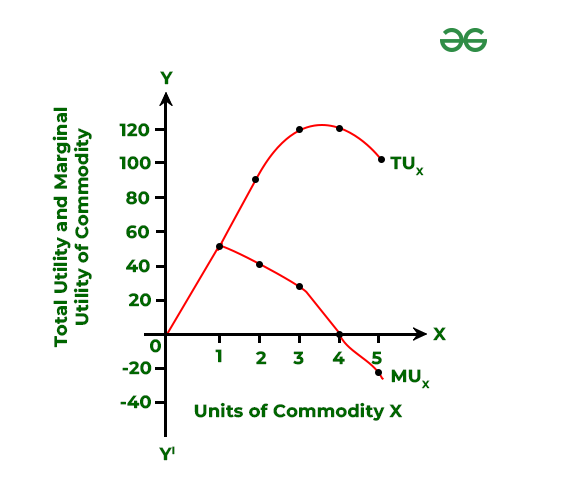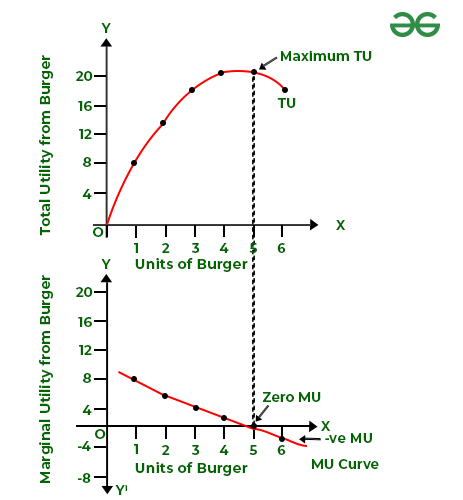Open in App
Not now

# What is Utility Analysis?|Total Utility and Marginal Utility

• Last Updated : 09 Feb, 2023

Consumers are the ones who make the majority of consumption decisions. A consumer is someone who buys goods and services to fulfil demands. He makes choices about the kinds of items to be bought to fulfil his desires. The primary goal is to maximise satisfaction from the goods and services he purchases with his income. To achieve the highest level of satisfaction, a consumer must follow certain rules or principles since resources are limited in nature in comparison to limitless demands. The two basic approaches for studying customer behaviour are the Cardinal Utility approach and Ordinal Utility Approach.

#### What is Cardinal Utility Approach?

People consume different goods and services to maximise their level of satisfaction. To achieve this, it is required to ascertain the level of satisfaction attained from a certain commodity. The Cardinal Utility Approach employs the concept of “Utility” to determine the level of satisfaction.

### What is Utility?

The characteristic of a good or service that allows it to fulfil the needs of consumers is comprehended as its “utility.” It is the fulfilment—actual or anticipated—that results from the consumption of a good or service. Utility is a relative concept, this means that it differs from individual to individual, from location to location, and from period to period. A utility is its ability to satisfy a need.

Simply put, Utility is the want satisfying power of a commodity.

It is considered to be measured in terms of cardinal numbers such as 1, 2, 3, 4, and so on. These are known as utils or units of utility. Thus, four utils are more significant than three utils, three utils are more significant than two utils, and so on.

#### How to measure Utility?

According to classical economics, utility can be measured similarly to how one would measure one’s height or weight. According to economists, the utility can be quantified in cardinal terms. It is possible to quantify the utility that an individual obtains through the consumption of commodities and services. However, there was no standardised method of calculating utility; so the economists came up with a hypothetical unit of measurement called Util.

Utils are imaginary and psychological units that are used to measure satisfaction obtained from the consumption of a certain quantity of a commodity.

Let’s say you just finished a cake and a bar of chocolate. You decide to label 15 utils as cake-derived utils. How many utils should be assigned to the chocolate now? Less than 15 utils would be given to chocolate if someone didn’t enjoy it as much. On the other hand, if one prefers chocolate more, then more than 15 utils will be assigned.

#### One more way to measure Utility

As utils vary from individual to individual, it cannot be taken as a standard unit for measurement. Therefore, various economists suggested that utility should be measured in monetary terms. Simply put, they suggested that utility can be measured in terms of price or money a consumer is willing to pay.

In the above example, let us assume that 1 util is equal to ₹1. Now, the utility derived from the consumption of cake will be ₹15, which is known as the value of utility in terms of money. The major advantage of using the monetary value of utility instead of utils is that monetary value allows easy comparison between the price paid for the commodity and the utility derived from it.

### 1. Total Utility

Total Utility determines the overall satisfaction obtained after consuming every single unit of that commodity. It is the total utility derived from the consumption of all units of a commodity.

For example, if 2 units of a commodity are consumed and the 1st unit provides 20 utils of satisfaction and the 2nd unit provides 14 utils of satisfaction, then the total utility will be 20+ 14 utils = 34 utils. If 3rd unit of a commodity is consumed and it provides 10 utils of satisfaction, then the total utility will be 20+14+10 = 44 utils.

Total Utility can be calculated as:

TUn = U1 + U2 + U3+…………………….+ Un

Where,

TUn = Total utility from n units of a given commodity

U1, U2, U3,……….Un = Utility derived from 1st, 2nd, 3rd,…………..nth unit

n = Number of units consumed

### 2. Marginal Utility

Marginal Utility is the utility obtained from the last unit of a product or service. It refers to the additional utility on account of the consumption of an additional unit of a commodity.

In the above example, when 3 units of a commodity provide the satisfaction of 44 utils, and 2 units of a commodity provide satisfaction of 34 utils, then the marginal utility will be 44 – 34 = 10 utils. The additional 10 utils derived from the 3rd unit are marginal utility.

MU can be calculated as:

MUn = TUn – TUn-1

Where,

MUn = Marginal utility from n units of a given commodity

TUn = Total utility from n units of a given commodity

TUn-1 = Total utility from n-1 units

n = Number of units consumed

#### One more way to calculate MU

MU is the change in TU caused by the consumption of one extra unit. MU can also be calculated when the change in units consumed is more than one.This results in another formula for calculating TU.

TUn = MU1 + MU2 + MU3+…………………….+ MUn, or

TU = ∑MU

The below table illustrates the estimation of MU and TU.

The above table shows that:

(i) MUn = TUn – TUn-1

Marginal utility of an nth unit of a commodity =Total utility from n units – Total utility from (n-1) units

(ii) TU = ΣMU

= 50 + 40 + 30 + 0 + (-20)

= 100### Relationship between TU and MU

The relationship between TU and MU can be explained with the help of the following schedule and diagram.Observation:

1. TU increases with an increase in the consumption of a commodity and as long as MU is positive. In this case, TU increases till 4th burger. Till 4 burgers, TU increases at a diminishing rate as MU from each successive burger diminishes.

2. When TU is at its maximum point, MU becomes zero; i.e., when the 5th burger is consumed. This point is known as the point of satiety.

3. Ultimately, when the consumption of a commodity is increased beyond the point of satiety, TU starts falling as MU becomes negative.

#### Basic Limitation of Utility Analysis

The fundamental problem in utility analysis is that it makes the incorrect assumption that utility can only be stated in cardinal numbers. it implies that utils like 1, 2, and 3 can never accurately represent usefulness. When two things are consumed concurrently, satisfaction can be compared at best. It is challenging to assess in figures. Due to this drawback, utility analysis is shown to have minimal application in describing consumer equilibrium.

#### What is Ordinal Utility Approach?

This approach states that utility can not be expressed in cardinal numbers like 1,2,3, and 4, rather it can only be ranked as high or low. The concept of cardinal utility was discarded by modern economists. According to them, utility is a psychological experience that cannot be quantified in absolute terms. They believe that consumers can order different combinations of goods and services to their preferences.

For example, if a consumer consumes two goods like Tea and Coffee, then he can say that:

1. He prefers tea over coffee;
2. He prefers coffee over tea;
3. Both are equally preferable and both of them provide the same level of satisfaction. This indicates that he is indifferent between tea and coffee.

My Personal Notes arrow_drop_up